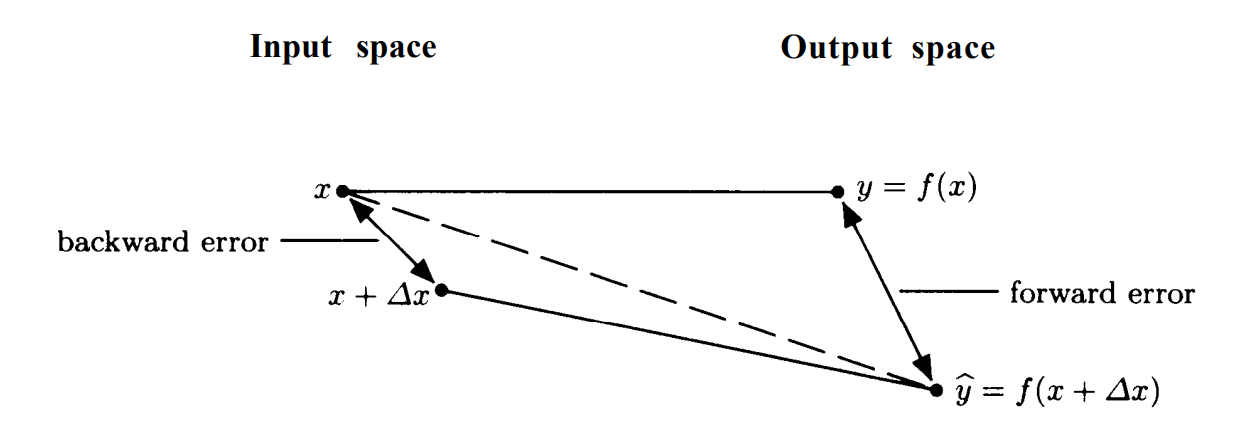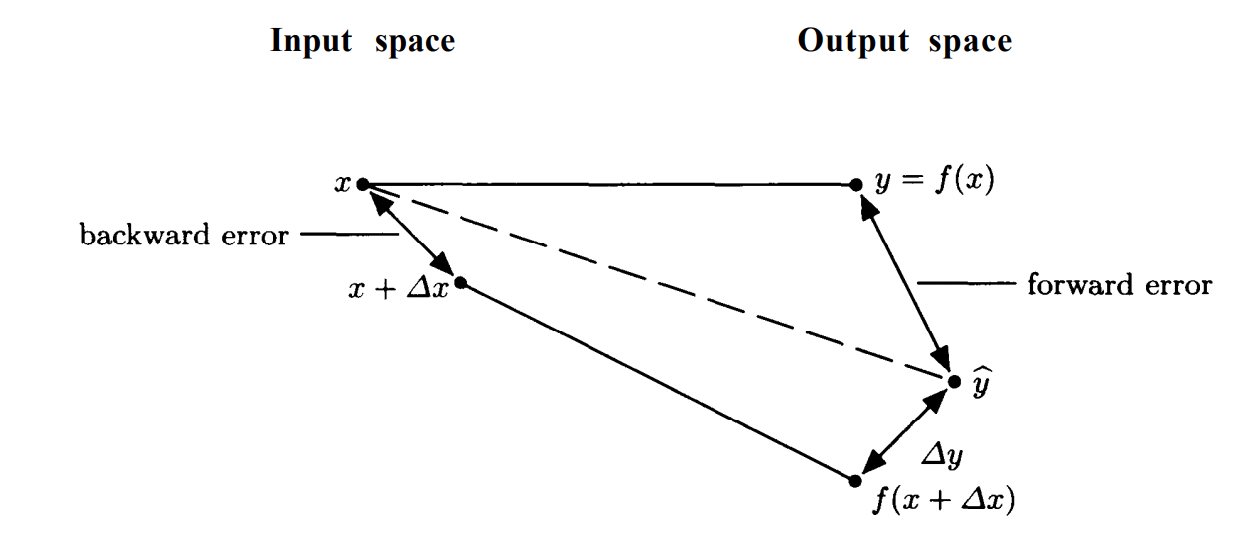## § A hacker's guide to numerical analysis

Life may toss us ill-conditioned problems, but it is too short to settle for unstable algorithms. - D.P. O'Leary

#### § Measures of error

If $x$ is a number and $\hat x$ is its approximation, then the are two notions of error:
1. absolute errror: $|x - \hat x|$.
2. relative error: $|x - \hat x|/|x|$.
Since the relative error is invariant under scaling $(x \mapsto \alpha x)$, we will mostly be interested in relative error.

#### § Significant digits

The significant digits in a number are the first nonzero digit and all succeeding digits. Thus 1.7320 has five significant digits. 0.0491 has only three significant digits. It is not transparent to me why this definition is sensible .

#### § Correct Significant digits --- a first stab

We can naively define $\hat x$ agrees to $x$ upto $p$ significant digits if $\hat x$ and $x$ round to the same number upto $p$ significant digits. This definition is seriously problematic. Consider the numbers:
• $x = 0.9949$, $x_1 = 1.0$, $x_2 = 0.99$, $x_3 = 0.9950$
• $y = 0.9951$, $y_1 = 1.0$, $y_2 = 1.0$, $y_3 = 0.9950$
Here, $y$ has correct one and three significant digits relative to $x$, but incorrect 2 significant digits, since the truncation at $x_2$ and $y_2$ do not agree even to the first significant digit.

#### § Correct Significant digits --- the correct definition

We say that $\hat x$ agress to $x$ upto $p$ significant digits if $|x - \hat x|$ is less than half a unit in the pth significant digit of $x$.

#### § Accuracy v/s precision

• Accuracy: absolute or relative error of a quantity.
• Precision: accuracy with which basic arithmetic +, -, *, / are performed. for floating point, measured by unit round-off (we have not met this yet).
Accuracy is not limited by precision : By using fixed precision arithmetic, we can emulate arbitrary precision arithmetic. The problem is that often this emulation is too expensive to be useful.

#### § Backward, Forward errors

Let $y = f(x)$, where $f: \mathbb R \rightarrow \mathbb R$. Let us compute $\hat y$ as an approximation to $y$, in an arithmetic of precision $u$. How do we measure the quality of $\hat y$?
1. In many cases, we maybe happy with an $\hat y$ such that the relative error between $y$ and $\hat y$ is equal to $u$: we did the best we can with the precision that was given. This is the forward error .
2. An alternative question we can ask is, for what $\delta x$ do we have that $\hat y = f(x + \delta x)$. That is, how far away from the input do we need to stray, to get a matching output? There maybe many such $\delta x$, so we ask for $\min |\delta x|$. We can divide this error by $x$ as a normalization factor. This is the backward error .There are two reasons we prefer backward error.
1. It converts error analysis into "data analysis". The data itself tends to be uncertain. If the error given by the backward analysis is smaller than the data uncertainty, then we can write off our error as being too small. Since for all we know, we have 'fixed' the uncertain data with our small error.
2. It reduces the question of error analysis into perturbation theory, which is very well understood for a large class of problems.

#### § Backward stable

A method for computing $y = f(x)$ is called backward stable if it produces a $\hat y$ with small backward error. That is, we need a small $\delta x$ such that $\hat y = f(x + \delta x)$.

#### § Mixed forward-backward error

We assume that addition and subtraction are backward stable, where $u$ is the number of significant digits to which our arithmetic operations can be performed:
$x \pm y = x(1 + \Delta) \pm y(1 + \Delta) \forall |\Delta| \leq u$
Another type of error we can consider is that of the form:
$\hat y + \delta y = f(x + \Delta x)$
That is, for a small perturbation in the output $(\delta y)$, we can get a backward error of $\delta x$. This is called as mixed forward backward error .We can say that an algorithm with mixed-forward-backward-error is stable iff:
\begin{aligned} &\hat y + \delta y = f(x + \Delta x) \\ &|\Delta y|/|\hat y| < \epsilon \\ &|\Delta x|/|\hat x| < \eta \\ &\text{\epsilon, \eta are small} \end{aligned}
This definition of stability is useful when rounding errors are the dominant form of errors.

#### § Conditioning

Relationship between forward and backward error is govered by conditioning : the sensitivity of solutions to perturbations of data. Let us have an approximate solution $\hat y = f(x + \delta x)$. Then:
\begin{aligned} &\hat y - y = f(x + \delta x) - f(x) = f'(x) \delta x + O((\delta x)^2) \\ &(\hat y - y)/y = (x f'(x)/f(x)) (\Delta x/x) + O((\Delta x)^2) \\ &(\hat y - y)/y = c(x) (\Delta x/x) + O((\Delta x)^2)\\ &c(x) \equiv |x f'(x)/f(x)| \end{aligned}
The quantity $c(x)$ measures the scaling factor to go from the relative change in output to the relative change in input. Note that this is a property of the function $f$, not any particular algorithm.

#### § Example: $\log x$

If $f(x) = \log x$, then $c(x) = |(x (\log x)') / \log x| = |1/\log x|$. This quantity is very large for $x \simeq 1$. So, a small change in $x$ can produce a drastic change in $\log x$ around $1$.
• Note the the absolute change is quite small: $\log(x + \delta x) \simeq \log x + \delta x/x$. However, relative to $\log x$, this change of $\delta x/x$ is quite large.

#### § Rule of thumb

$\text{forward error} \lesssim \text{condition number} \times \text{backward error}$
• Glass half empty: Ill-conditioned problems can have large forward error.
• Glass half full: Well-conditioned problems do not amplify error in data.

#### § Forward stable

If a method produces answers with forward errors of similar magnitude to those produced by a backward stable method, then it is called forward stable. Backward stability implies forward stability, but not vice-versa (TODO: why?)

#### § Cancellation

Consider the following program:
#include <cmath>
#include <stdio.h>

int main() {
double x = 12e-9;
double c = cos(x);
double one_sub_c = 1 - c;
double denom = x*x;
double yhat = one_sub_c / denom;

printf("x:         %20.16f\n"
"cx:        %20.16f\n"
"one_sub_c: %20.16f\n"
"denom:     %20.16f\n"
"yhat:      %20.16f\n",
x, c, one_sub_c, denom, yhat);
}

which produces the output:
x:           0.0000000120000000
cx:          0.9999999999999999
one_sub_c:   0.0000000000000001
denom:       0.0000000000000001
yhat:        0.7709882115452477

This is clearly wrong , because we know that $(1-\cos x)/x^2) \leq 1/2$. The reason for this terrible result is that:
• we know $\cos x$ to high accuracy, since $x$ was some fixed quantity.
• $1 - \cos x$ converted the error in $\cos x$ into its value .
• $1 - \cos x$ has only one significant figure.
• This makes it practically useless for anything else we are interested in doing.
In general:
\begin{aligned} &x \equiv 1 + \epsilon \text{error of order \epsilon} \\ &y \equiv 1 - x = \epsilon \text{value of order \epsilon} \\ \end{aligned}
That is, subtracting values close to each other (in this case, $1$ and $x$) converts error order of magnitude into value order of magnitude . Alternatively, it brings earlier errors into promience as values.

#### § Analysis of subtraction

We can consider the subtraction:
\begin{aligned} &x = a - b; \hat x = \hat a - \hat b \\ &\hat a = a(1 + \Delta a) \\ &\hat b = b(1 + \Delta b) \\ &\left| \frac{x - \hat x}{x} \right| \\ &= \left| \frac{-a\Delta a - b\Delta b}{a - b} \right| \\ &= \frac{|-a\Delta a - b\Delta b|}{|a - b|} \\ &= \frac{|a\Delta a + b\Delta b|}{|a - b|} \\ &\leq \frac{\max(|\Delta a|, |\Delta b|) (|a| + |b|)}{|a - b|} \end{aligned}
This quantity will be large when $|a - b| \ll |a| + |b|$: that is, when there is heavy cancellation in the subtraction to compute $x$.

#### § Underflow

#include <cmath>
#include <stdio.h>

int main() {
double x = 1000;
for(int i = 0; i < 60; ++i) {
x = sqrt(x);
}
for(int i = 0; i < 60; ++i) {
x = x*x;
}
printf("x: %10.20f\n", x);
}

This produces the output:
./sqrt-pow-1-12
...
x: 1.00000000000000000000

That is, even though the function is an identity function, the answer collapses to 1. What is happening?

#### § Computing $(e^x - 1)/x$

One way to evaluate this function is as follows:
double f(double x) { return x == 0 ? 1 : (pow(M_E, x) - 1) / x; }

This can suffer from catastrophic cancellation in the numerator. When $x$ is close to $0$, $e^x$ is close to 1, and $e^x - 1$ will magnify the error in $e^x$.
double f(double x) {
const double y = pow(M_E, x);
return y == 1 ? 1 : (y - 1) / log(y);
}

This algorithm seems crazy, but there's insight in it. We can show that the errors cancel! The idea is that neither $(y - 1)$ nor $\log y$ are particularly good, the errors accumulated in them almost completely cancel out, leaving out a good value:
$\text{assume \hat y = 1} \\ 1 = \hat y \equiv e^x(1 + \delta) \\ \log 1 = \log (e^x ) + \log(1 + \delta) \\ x = -\log(1 + \delta) \\ x = -\delta + O(\delta^2)$
If $\hat y \neq 1$:
$\hat f = (\hat y - 1)/\log{\hat y} = (1+\epsilon_3)(\hat y - 1)(1 + \epsilon+1)/(\log \hat y(1 + \epsilon_2))$

#### § IEEE floating point fun: +0 and -0 for complex analysis

Rather than think of +0 and -0 as distinct numerical values, think of their sign bit as an auxiliary variable that conveys one bit of information (or misinformation) about any numerical variable that takes on 0 as its value.
We have two types of zeroes, +0 and -0 in IEEE-754. These are used in some cases. The most famous is that $1/+0 = +\infty$, while $1/-0 = -\infty$. Here, we proceed to discuss some complex-analytic considerations.
Therefore. implementers of compilers and run-time libraries bear a heavy burden of attention to detail if applications programmers are to realize the full benefit of the IEEE style of complex arithmetic. That benefit deserves Some discussion here if only to reassure implementers that their assiduity will be appreciated.
$\sqrt{-1 + 0 i} = +0 + i \\ \sqrt{-1 - 0 i} = +0 - i \\$
These will ensure that $\sqrt{z*} = (\sqrt{z})*$:
$\texttt{copysign}(1, +0) = +1 \\ \texttt{copysign}(1, -0) = -1 \\$
These will ensure that $\texttt{copysign}(x, 1/x) = x$ when $x = \pm \infty$. An example is provided where the two limits:
\begin{aligned} &f(x + i0) = \lim_{y \rightarrow 0-} f(x + i y) \\ &f(x + i0) = \lim_{y \rightarrow 0-} f(x + i y) \\ \end{aligned}

#### § Complex-analytic considerations

The principal branch of a complex function is a way to select one branch of a complex-function, which tends to be multi-valued. A classical example is the argument function, where $\arg(r e^{i \theta} = \theta$. However, this is ambiguous: we can map $\theta \mapsto \theta + 2 \pi$ and still have the same complex number. So, we need to fix some standard. We usually pick the "branch" where $0 \leq \theta < 2 \pi$. In general, we need to carefully handle what happens to the function at the discontinuity.
What deserves to be undermined is blind faith in the power of Algebra. We should not believe that the equivalence class of expressions that all describe the same complex analytic function can be recognized by algebraic means alone, not even if relatively uncomplicated expressions are the only ones considered.